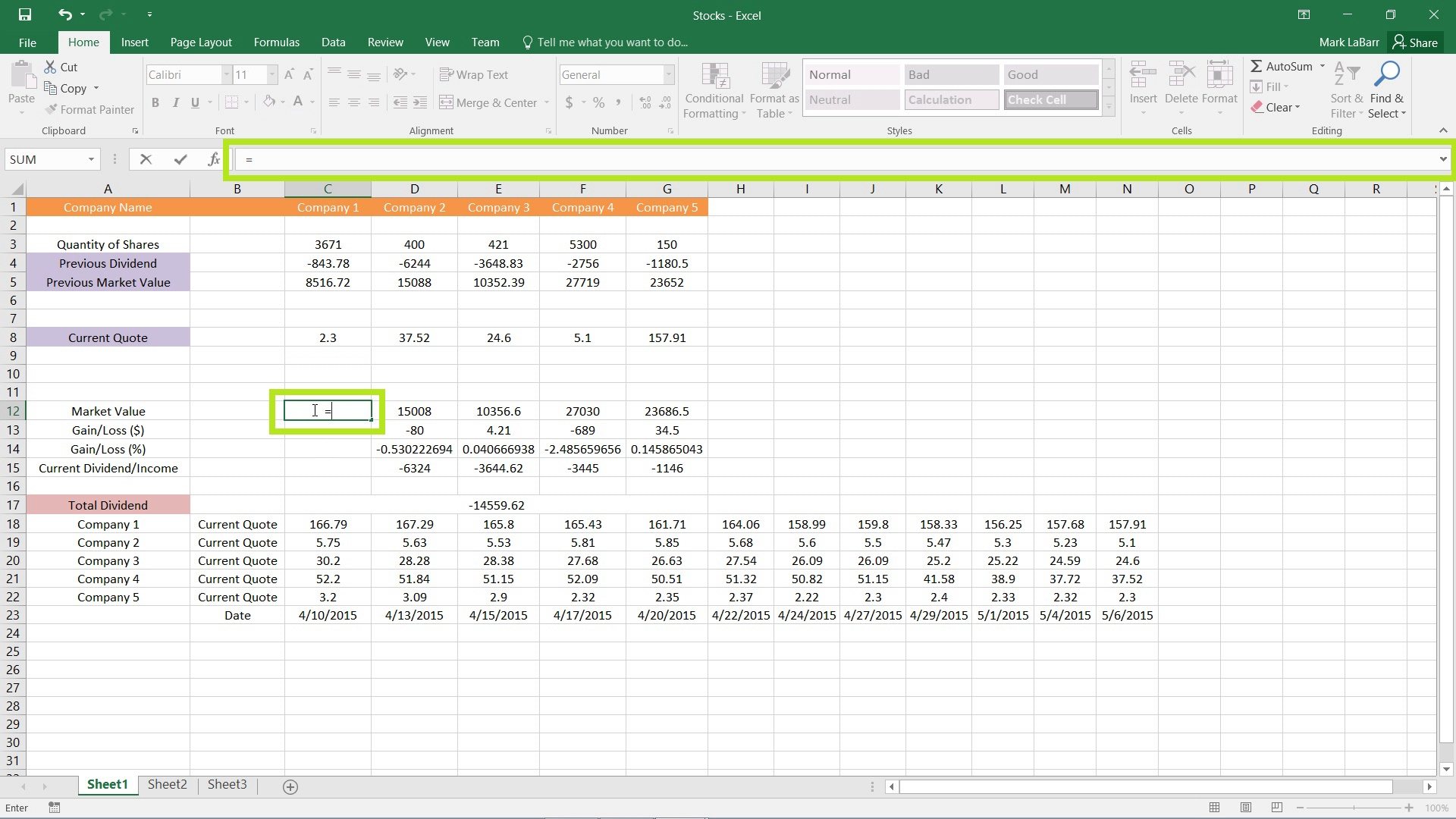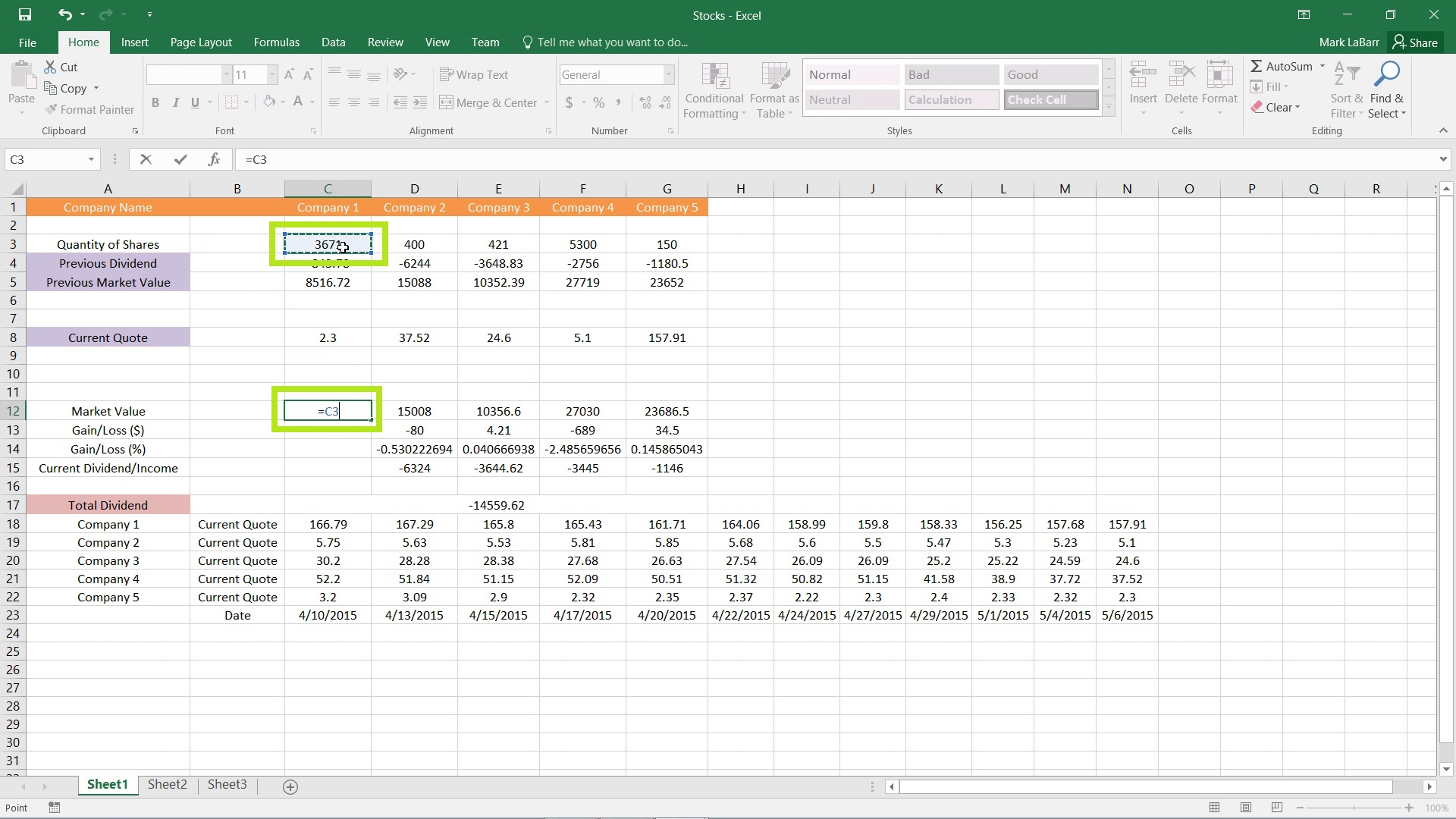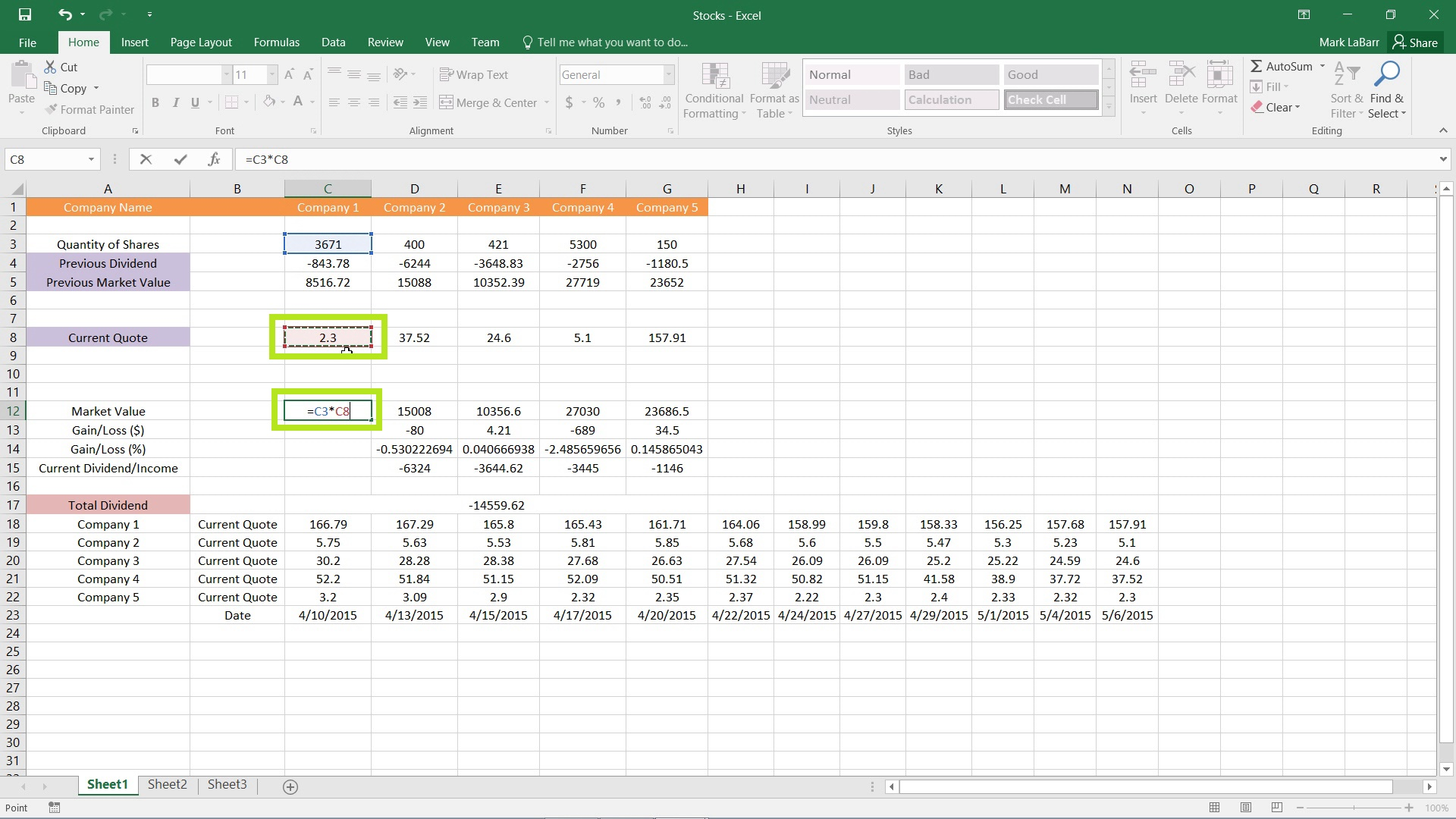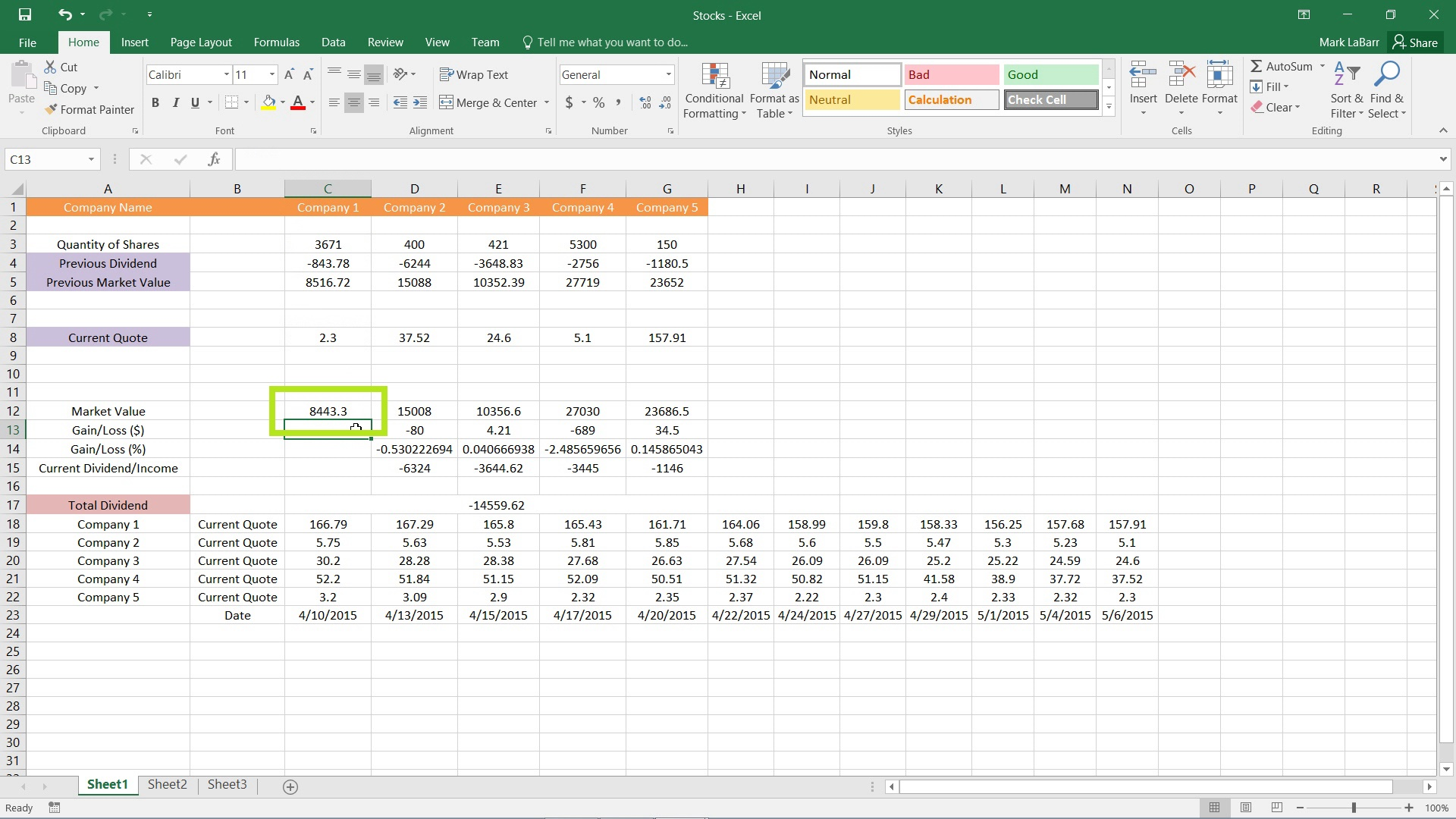## Creating Basic Formulas in Excel 2016

In this tutorial, we’re going to show you how to create basic formulas in Excel 2016.

To get started, choose a cell in which you want the formula and type “=” to tell Excel that you are going to type in a formula. You may either type directly in the cell or the formula bar highlighted below.To use a cell in the formula to be included in the calculations, simply click on the cell or type its address in.The formula used for the example uses a multiplication operation. To multiply, type “*” (asterisk symbol) then click the next cell you want to multiply the first by.When you have finished typing in your formula, hit enter or click a different cell to have Excel calculate the value.